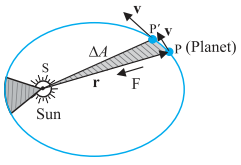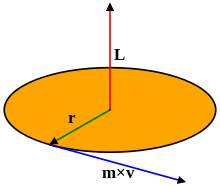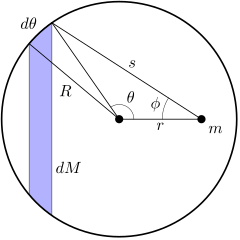# Topic: Gravitation (Test 1)

Topic: Gravitation
Q.1
According to Kepler’s Law of orbits:
A. All planets move in elliptical orbits with the Sun situated at one of the foci of the ellipse
B. All planets move in parabolic orbits with the Sun situated at one of the foci of the parabola.
C. All planets move in parabolic orbits with the Earth situated at one of the foci of the parabola.
D. All planets move in elliptical orbits with the earth situated at one of the foci of the ellipse.
Answer : Option A
Explaination / Solution:

The orbit of a planet around the Sun (or of a satellite around a planet) is not a perfect circle. It is an ellipse—a “flattened” circle. The Sun (or the centre of the planet) occupies one focus of the ellipse. A focus is one of the two internal points that help determine the shape of an ellipse. The distance from one focus to any point on the ellipse and then back to the second focus is always the same.

Workspace
Report
Q.2
According to Kepler’s Law of areas,
A. The line that joins any planet to the sun sweeps equal areas in equal intervals of time.
B. Only the line that joins mars to the earth sweeps equal areas in equal intervals of time
C. The line that joins any planet to the earth sweeps equal areas in equal intervals of time
D. Only the line that joins earth to the sun sweeps equal areas in equal intervals of time
Answer : Option A
Explaination / Solution:

Kepler law of areas describes the speed of a planet travelling in an elliptical orbit around the sun. A planet’s orbital speed changes, depending on how far it is from the Sun. The closer a planet is to the Sun, the stronger the Sun’s gravitational pull on it, and the faster the planet moves. The farther it is from the Sun, the weaker the Sun’s gravitational pull, and the slower it moves in its orbit. So, statement of Kepler's law of area is "The line joining the sun to the planet sweeps out equal areas in equal interval of time". i.e. areal velocity is constant.

Workspace
Report
Q.3
According to Kepler’s Law of periods, The _______________ of the time period of revolution of a planet is proportional to the cube of the ___________ of the ellipse traced out by the planet
A. cube, semi-major axis
B. square, semi-major axis
C. cube, semi-major axis
D. square, semi-minor axis
Answer : Option B
Explaination / Solution:

Kepler's 3rd Law: T= a3. Kepler's 3rd law is a mathematical formula. It means that if you know the period of a planet's orbit (T = how long it takes the planet to go around the Sun), then you can determine that planet's distance from the Sun (a is the length of the semimajor axis of the planet's orbit)

Workspace
Report
Q.4

The area A swept out by a planet of mass m in time interval t is related to the angular momentum by:

A. ΔA/Δt = 2L / m
B. ΔA/Δt = L / (2m)
C. ΔA/Δt = gL / (2 m)
D. ΔA/Δt = GL / (2 m)
Answer : Option B
Explaination / Solution:The law of areas can be understood as the consequence of conservation of angular momentum which is valid for any central for A central force is such that the force on the planet is along the vector joining the sun and the planet. Let the sun be at the origin and let the position and momentum of the planet be denoted by r and p  respectively. Then the area swept out by the planet of mass m in the time interval Δt is given by ΔA = ½  (r × vΔt).

Hence ΔA/Δt    =½ (r × p)/m, (since  v=p/m)

=    L / (2 m)

where v is the velocity,  where v is the velocity,  L is the angular
momentum equal to   ( r  ×  p  ).  For a central force, which is directed along r, L is a constant

Workspace
Report
Q.5

Newton’s law of universal gravitation states that the gravitational force of attraction between any two particles of masses m1 and m2 separated by a distance r has the magnitude

A. F=m1m2r2
B. F=Gm2r2
C. F=Gm1r2
D. F=Gm1m2r2
Answer : Option D
Explaination / Solution:

According to Newton's law of universal gravitation, the force of attraction between two objects is directly proportional to the product of two masses and inversely proportional to the square of the distance between them

Workspace
Report
Q.6
The direction of the universal gravitational force between particles of masses m1 and m2 is:
A. towards
B. towards on  and  towards  on .
C.
towards the center of the earth

D. towards
Answer : Option B
Explaination / Solution:

since gravitational force is attractive in nature, so if there are two particles of m& m2 .

So One Force will be on m1 which will be directed towards m 2. i.e

And other force wiil be on m2 which will be directed towards m 1 i.e

Clearly, It can be seen that  Because thsese forces are attracted to each other and direction of each force is opposite to other one.

Workspace
Report
Q.7
A ‘central’ force is always directed
A. at a fixed angle to the position vector of the point of application of the force with respect to the fixed point
B. along the position vector of the point of application of the force with respect to the fixed point
C. at a varying angle to the position vector of the point of application of the force with respect to the fixed point
D. perpendicular to the position vector of the point of application of the force with respect to the fixed point
Answer : Option B
Explaination / Solution:The motion of a particle under a central force F always remains in the plane defined by its initial position and velocity.This may be seen by symmetry. Since the position r, velocity v and force F all lie in the same plane, there is never an acceleration perpendicular to that plane, because that would break the symmetry between "above" the plane and "below" the plane.

To demonstrate this mathematically, it suffices to show that the angular momentum of the particle is constant. This angular momentum L is defined by the equation

Workspace
Report
Q.8

The gravitational force on point mass m1 due to point mass m2, m3 & m4 is:

A. the difference of gravitational forces exerted by  and
B. the scalar sum of the gravitational forces exerted by  and
C. the difference of gravitational forces exerted by  and
D. the vector sum of the gravitational forces exerted by  ,  and
Answer : Option D
Explaination / Solution:

According to properties of gravitational force: force between the particles is independent of the presence or absence of other particles; so the principle of superposition is valid i.e. force on a particle due to a number of particles is the resultant of forces due to individual particles i.e.
Workspace
Report
Q.9
To find the resultant gravitational force acting on the particle m due to a number of masses we need to use:
A. the principle of no action
B. the principle of least action
C. the principle of superposition
D. the principle of maximal action
Answer : Option C
Explaination / Solution:

According to properties of gravitational force, gravitational force between the particles is independent of the presence or absence of other particles; so the principle of superposition is valid i.e. force on a particle due to number of particles is the resultant of forces due to individual particles i.e.
Workspace
Report
Q.10
The force of attraction between a hollow spherical shell of uniform density and a point mass situated outside is just as if the entire mass of the shell is
A. equally concentrated at three points on a triangle of the shell.
B. concentrated at the centre of the shell.
C. equally concentrated at opposite ends of the diameter of the sphere
D. equally concentrated at four points on a square of the shell.
Answer : Option B
Explaination / Solution:According to Shell's theorem, If a particle of mass m is located outside a spherical shell of mass M at, for instance, point P, the shell attracts the particle as though the mass of the shell were concentrated at its centre. Thus, as far as the gravitational force acting on a particle outside the shell is concerned, a spherical shell acts no differently from the solid spherical distributions of mass.

Workspace
Report